CATEGORIES:

RATE OF A CHEMICAL REACTION. CHEMICAL EQUILIBRIUM

The rate of a chemical reaction can be considered as a change of the amount of reactants or products per a unit time:

v =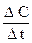It is affected by the nature of reactants, their concentrations, temperature, and the presence of a catalyst.

Concentration dependence:

For a simple reaction m A + n B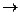p C

v = k [A]m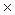[B]n (law of mass action)

where k - rate constant; m and n - reaction orders.

For a simple reaction m and n are stoichiometric coefficients; for a multistep reaction reaction orders are determined experimentally from the step with the lowest rate.

Temperature dependence:

v(T2) = v(T1)g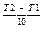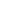(Vant Hoff’s equation)where g - temperature coefficient.

For majority of homogenous reactions the evaluation of temperature by 10 degrees provides the 2-4 times evaluation of the rate of the reaction. This significant growth of the rate is due to the evaluation of the number of active molecules in a geometrical progression.

The extra energy of molecules which provokes their change and the appearance of new substances is called the activation energy.

Catalyst dependence:

The rate of a chemical reaction grows in the presence of a catalyst. The latter causes unstable intermediates (activated complexes) to appear whose decomposition leads to the formation of products. As a result the activation energy of a chemical reaction decreases, and the rate of the reaction increases.

Reversibility of chemical reactions:

For reversible reactions when the rates of both forward and reverse processes become the same, a state of a chemical equilibrium sets in.

The state of a chemical equilibrium can be described in terms of equilibrium constant K: for a reaction m A + n Bp C

K =When conditions (temperature, pressure, concentration of one of the reactants) of the reversible reaction change, the rates of the forward and reverse processes change differently and the chemical equilibrium shifts according to Le Chatelier’s principle:

If any change of conditions is imposed on a system in equilibrium, the equilibrium will shift in such a way as to counteract the imposed change.

Date: 2015-01-12; view: 933

 <== previous page | next page ==> EXPERIMENTS in General and Inorganic Chemistry | QUESTIONS AND PROBLEMS
doclecture.net - lectures - 2014-2022 year. Copyright infringement or personal data (0.002 sec.)